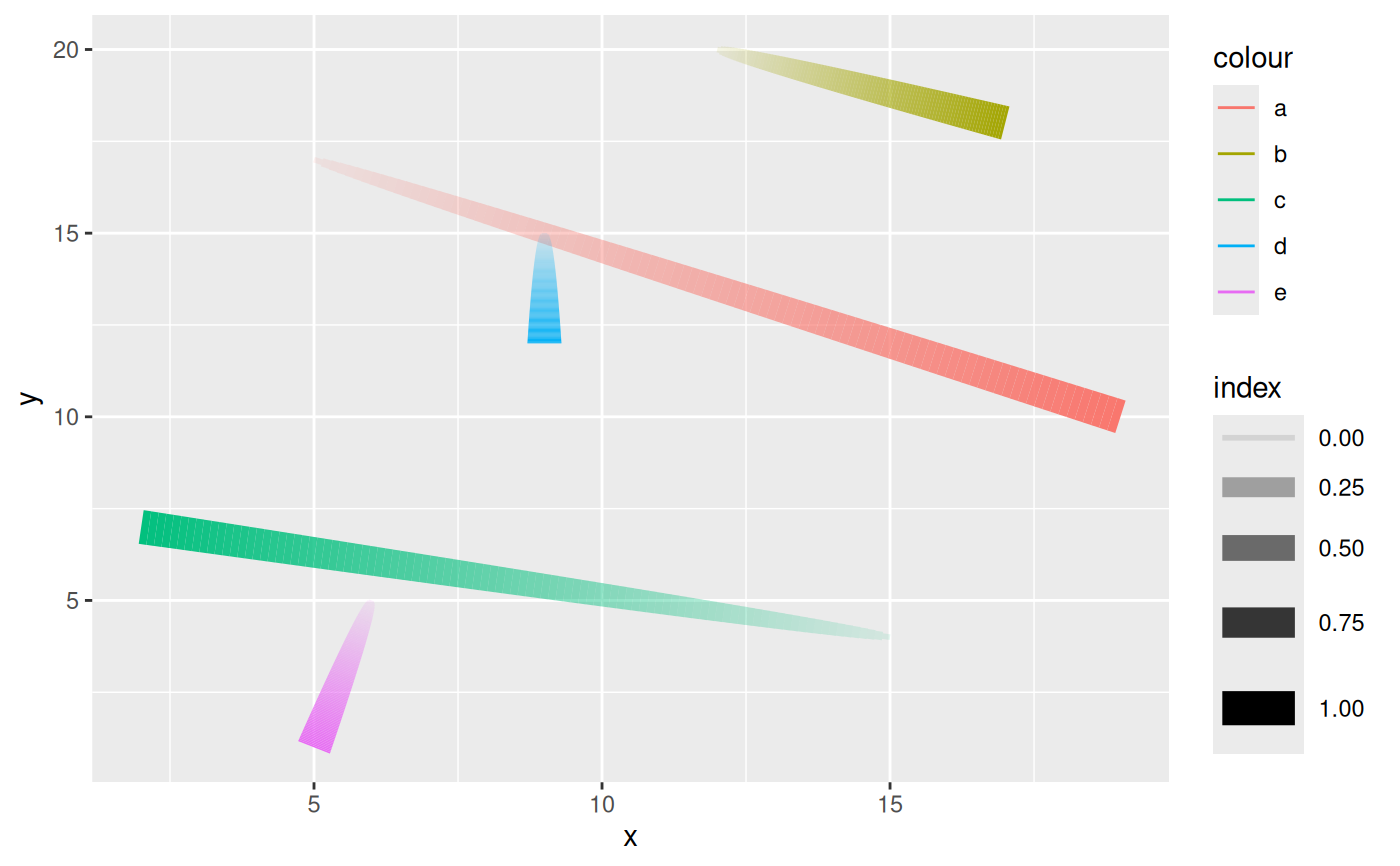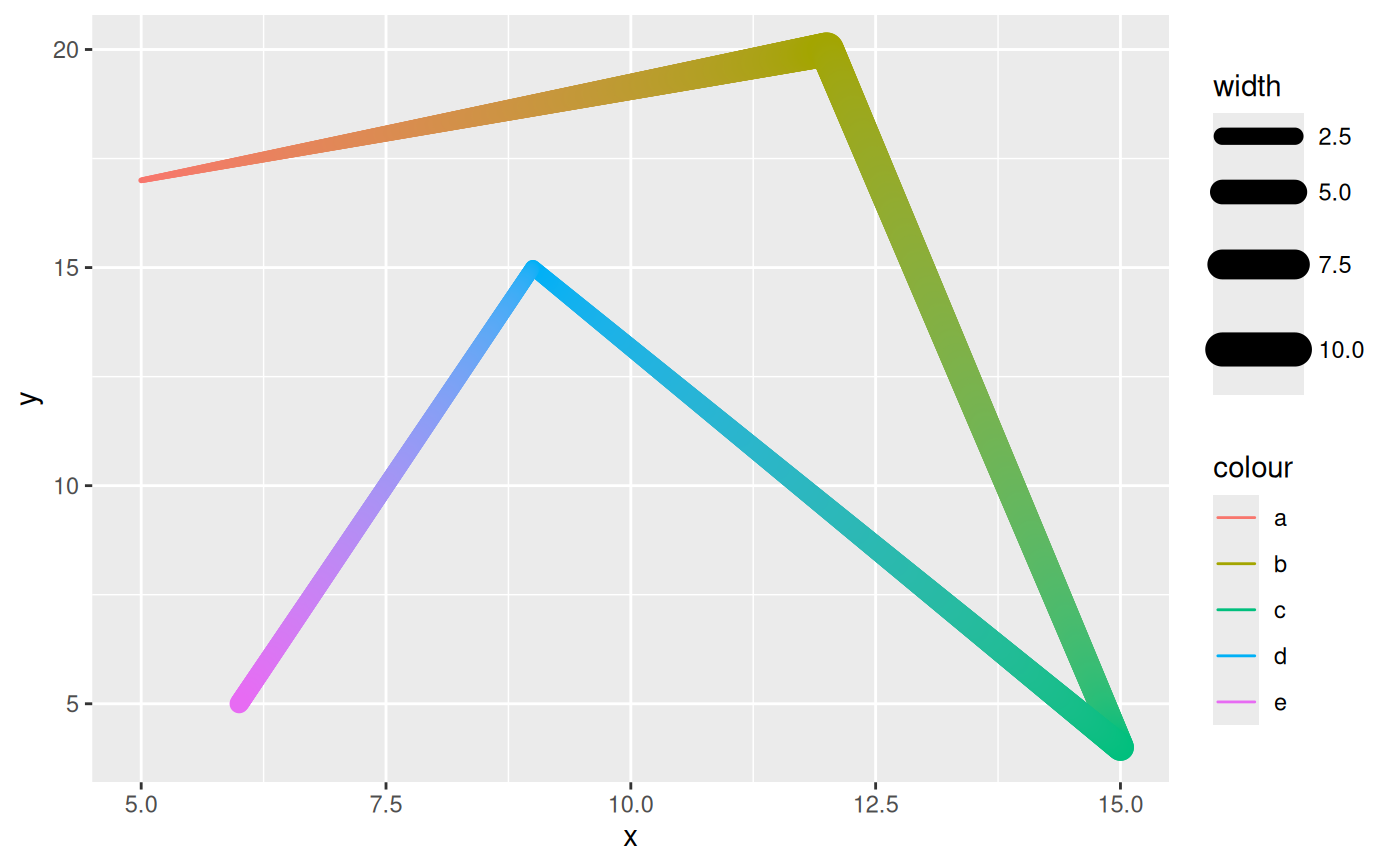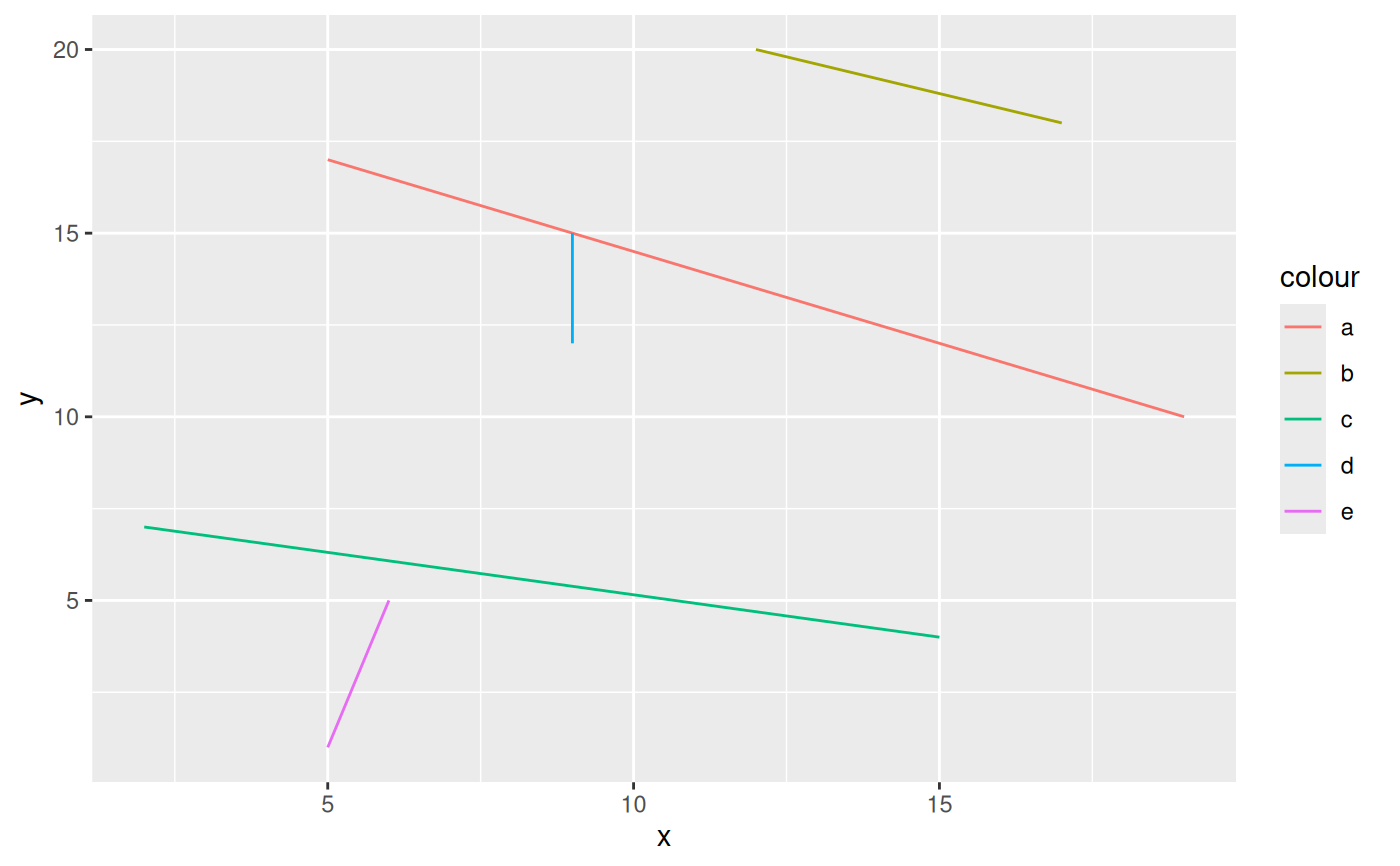This set of geoms makes it possible to connect points using straight lines. Before you think ggplot2::geom_segment() and ggplot2::geom_path(), these functions have some additional tricks up their sleeves. geom_link connects two points in the same way as ggplot2::geom_segment() but does so by interpolating multiple points between the two. An additional column called index is added to the data with a sequential progression of the interpolated points. This can be used to map color or size to the direction of the link. geom_link2 uses the same syntax as ggplot2::geom_path() but interpolates between the aesthetics given by each row in the data.

stat_link(
mapping = NULL,
data = NULL,
geom = "path",
position = "identity",
na.rm = FALSE,
show.legend = NA,
n = 100,
inherit.aes = TRUE,
...
)

mapping = NULL,
data = NULL,
geom = "path_interpolate",
position = "identity",
na.rm = FALSE,
show.legend = NA,
n = 100,
inherit.aes = TRUE,
...
)

mapping = NULL,
data = NULL,
position = "identity",
arrow = NULL,
lineend = "butt",
na.rm = FALSE,
show.legend = NA,
inherit.aes = TRUE,
n = 100,
...
)

mapping = NULL,
data = NULL,
position = "identity",
arrow = NULL,
lineend = "butt",
na.rm = FALSE,
show.legend = NA,
inherit.aes = TRUE,
n = 100,
...
)

mapping = NULL,
data = NULL,
stat = "identity",
position = "identity",
...,
arrow = NULL,
arrow.fill = NULL,
lineend = "butt",
linejoin = "round",
na.rm = FALSE,
show.legend = NA,
inherit.aes = TRUE
)

## Arguments

mapping

Set of aesthetic mappings created by aes(). If specified and inherit.aes = TRUE (the default), it is combined with the default mapping at the top level of the plot. You must supply mapping if there is no plot mapping.

data

The data to be displayed in this layer. There are three options:

If NULL, the default, the data is inherited from the plot data as specified in the call to ggplot().

A data.frame, or other object, will override the plot data. All objects will be fortified to produce a data frame. See fortify() for which variables will be created.

A function will be called with a single argument, the plot data. The return value must be a data.frame, and will be used as the layer data. A function can be created from a formula (e.g. ~ head(.x, 10)).

geom

The geometric object to use to display the data, either as a ggproto Geom subclass or as a string naming the geom stripped of the geom_ prefix (e.g. "point" rather than "geom_point")

position

Position adjustment, either as a string naming the adjustment (e.g. "jitter" to use position_jitter), or the result of a call to a position adjustment function. Use the latter if you need to change the settings of the adjustment.

na.rm

If FALSE, the default, missing values are removed with a warning. If TRUE, missing values are silently removed.

show.legend

logical. Should this layer be included in the legends? NA, the default, includes if any aesthetics are mapped. FALSE never includes, and TRUE always includes. It can also be a named logical vector to finely select the aesthetics to display.

n

The number of points to create for each segment

inherit.aes

If FALSE, overrides the default aesthetics, rather than combining with them. This is most useful for helper functions that define both data and aesthetics and shouldn't inherit behaviour from the default plot specification, e.g. borders().

...

Other arguments passed on to layer(). These are often aesthetics, used to set an aesthetic to a fixed value, like colour = "red" or size = 3. They may also be parameters to the paired geom/stat.

stat

The statistical transformation to use on the data for this layer, either as a ggproto Geom subclass or as a string naming the stat stripped of the stat_ prefix (e.g. "count" rather than "stat_count")

arrow

Arrow specification, as created by grid::arrow().

lineend

Line end style (round, butt, square).

arrow.fill

fill colour to use for the arrow head (if closed). NULL means use colour aesthetic.

linejoin

Line join style (round, mitre, bevel).

## Aesthetics

geom_link understand the following aesthetics (required aesthetics are in bold):

• x

• y

• xend

• yend

• color

• size

• linetype

• alpha

• lineend

geom_link2 understand the following aesthetics (required aesthetics are in bold):

• x

• y

• color

• size

• linetype

• alpha

• lineend

## Computed variables

x, y

The interpolated point coordinates

index

The progression along the interpolation mapped between 0 and 1

## Examples

# Lets make some data
lines <- data.frame(
x = c(5, 12, 15, 9, 6),
y = c(17, 20, 4, 15, 5),
xend = c(19, 17, 2, 9, 5),
yend = c(10, 18, 7, 12, 1),
width = c(1, 10, 6, 2, 3),
colour = letters[1:5]
)

ggplot(lines) +
geom_link(aes(x = x, y = y, xend = xend, yend = yend, colour = colour,
alpha = stat(index), size = after_stat(index)))ggplot(lines) +
geom_link2(aes(x = x, y = y, colour = colour, size = width, group = 1),
lineend = 'round', n = 500)# geom_link0 is simply an alias for geom_segment to put the link geoms in
# line with the other line geoms with multiple versions. index is not
# available here
ggplot(lines) +
geom_link0(aes(x = x, y = y, xend = xend, yend = yend, colour = colour))